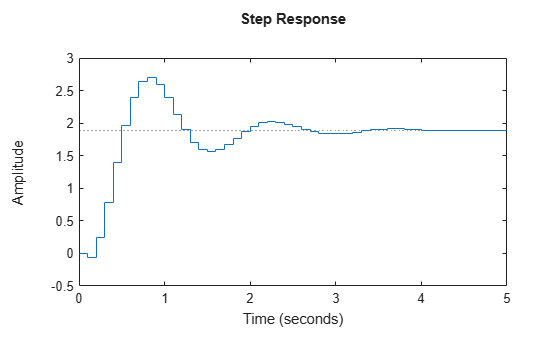# Time-Domain Responses of Discrete-Time Model

This example shows how to obtain a step-response plot and step-response data for a discrete-time dynamic system model. Obtaining time-domain responses of discrete-time models is the same as for continuous-time models, except that the time sample points are limited by the sample time `Ts` of the model.

You can use the techniques of this example with commands such as `impulse`, `initial`, `impulseplot`, and `initialpot` to obtain time-domain responses of discrete-time models.

Create a discrete-time transfer function model and plot its response to a step input at $t$ = 0.

```H = tf([-0.06,0.4],[1,-1.6,0.78],0.1); step(H)```For discrete-time models, `step` plots the response at multiples of the sample time, assuming a hold between samples.

Compute the step response of `H` between 0.5 and 2.5 seconds.

`[y,t] = step(H,0.5:0.1:2.5);`

When you specify a time vector for the response of a discrete-time model, the time step must match the sample time `Ts` of the discrete-time model. The vector `t` contains the time points between 0.5 and 2.5 seconds, at multiples of the sample time of `H`, 0.1 s. The vector `y` contains the corresponding step response values.